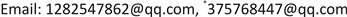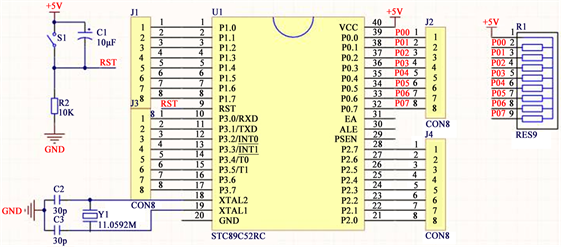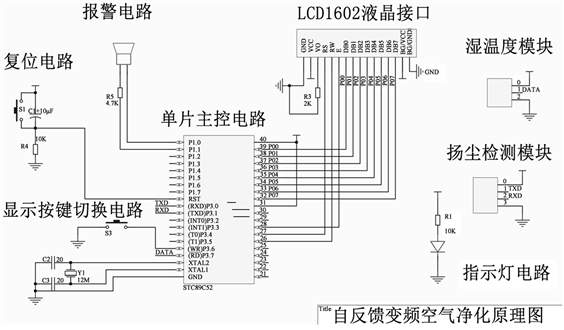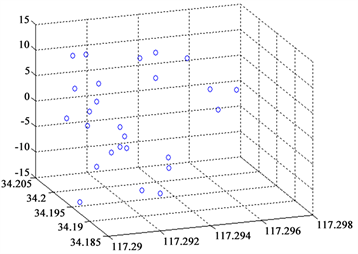﻿ 最小二乘多元线性回归及Mie光散射便携式扬尘粒子监测系统 Least Square Multiple Linear Regression and Mie Light Scattering of Portable Dust Particle Monitoring System

Computer Science and Application
Vol. 08  No. 09 ( 2018 ), Article ID: 26859 , 7 pages
10.12677/CSA.2018.89154

Least Square Multiple Linear Regression and Mie Light Scattering of Portable Dust Particle Monitoring System

Peng Liu, Xunguang Ju*, Jingzhou Han, Wei Dai

Information and Electrical Engineering College, Xuzhou Institute of Technology, Xuzhou JiangsuReceived: Aug. 29th, 2018; accepted: Sep. 12th, 2018; published: Sep. 19th, 2018ABSTRACT

Based on the Least Square Multiple Linear Regression (LSMLR) and Mie light scattering, a portable dust particle online monitoring system was designed. LSMLR was used to linearly compensate the data accuracy deviation. This solved the problem of low sensitivity of PM 2.5/10 particle concentration monitoring and provided data evidence and decision-making advice for environmental supervision and evaluation.

Keywords:Mie Light Scattering, Least Square Multiple Linear Regression, Dust Particle, Correct Deviation, Photoelectric Detection1. 引言

2. 设计方案

3. 工作原理

3.1. 基本原理简介

3.1.1. SDS011激光传感器原理

$F=N\frac{{\lambda }^{2}}{8{\text{π}}^{2}}\underset{D=\stackrel{¯}{w}}{\overset{D=\stackrel{¯}{w}}{\int }}\underset{\theta =\varphi -\beta }{\overset{\theta =\varphi +\beta }{\int }}\left[{i}_{1}\left(D,\theta ,m\right)+{i}_{2}\left(D,\theta ,m\right)\right]w\left(\theta ,\varphi \right){F}_{N}\left(D\right)\text{d}D\text{d}\theta$ (1)

3.1.2. 采用最小二乘和多元回归分析  修正后的测量结果校准方程

1) 相对湿度

DHT11可以通过DATA数据总线直接输出数字量湿度值。该湿度值称为“相对湿度”，需要进行线性补偿和温度补偿后才能得到较为准确的湿度值，湿度的非线性补偿如图1所示。为获得较为准确的测量数值，可以采用式(2)进行信号转换。

${\text{RH}}_{linear}={C}_{1}+{C}_{2}\cdot S{O}_{RH}+{C}_{3}\cdot S{O}_{\text{RH}}{}^{2}\left(\text{%RH}\right)$ (2)

2) 温度

$T={d}_{1}+{d}_{2}\cdot S{O}_{T}$ (3)Figure 1. STC 89C 52 pin diagramTable 1. Humidity compensation coefficient of temperature signal

3) 湿度信号的温度补偿

${\text{RH}}_{ture}=\left(T-25\right)\left({t}_{1}+{t}_{2}S{O}_{\text{RH}}\right)+{\text{RH}}_{linear}$ (4)

4) 湿温度信号对PM 2.5浓度补偿

${C}_{\text{PM}2.5ture}={C}_{\text{PM2}\text{.5}}-1.662T+2.431{\text{RH}}_{linear}+89.49$ (5)

3.2. 电路原理图

3.2.1. 扬尘粒子信号采集装置

5001-S03D是一款激光数字式粒子传感器，其采用光散射原理，激光在颗粒物上产生散射光，由光电接收器件转变为电信号，再通过特定算法计算得出PM 2.5和PM 10质量浓度、PM 0.3~PM 2.5粒子个数、PM 2.5~PM 10粒子个数。

3.2.2. 温度湿度信号采集装置

DHT11数字温湿度传感器是一款含有已校准数字信号输出的温湿度复合传感器。它应用专用的数字模块采集技术和温湿度传感技术，确保产品具有极高的可靠性与卓越的长期稳定性。传感器包括一个电阻式感湿元件和一个NTC测温元件，并与一个高性能8位单片机相连接。因此该产品具有品质卓越、超快响应、抗干扰能力强、性价比极高等优点。每个DHT11传感器都在极为精确的湿度校验室中进行校准。

3.2.3. 控制终端电路

3.2.4. 功能实现原理Table 3. Temperature compensation coefficient table of humidity signalFigure 2. Detection display schematic

3.2.5. 系统误差分析

4. 技术关键

5. 产品参数结果Figure 3. PM 2.5 concentration error

6. 创新点及应用

6.1. 创新点

1) 监测装置较小便于携带，可用来监测不同高度不同条件下的扬尘粒子，提高数据采集可靠性和系统的可扩展性

2) 基于物理上的Mie散射理论，实现不同条件下粒子含量测量，探析扬尘粒子的分布规律。

3) 应用最小二乘算法和多元线性回归，结合湿温度数据，对监测结果进行线性补偿，提高监测结果的准确性，使得低成本的监测技术更加准确。

6.2. 应用

Least Square Multiple Linear Regression and Mie Light Scattering of Portable Dust Particle Monitoring System[J]. 计算机科学与应用, 2018, 08(09): 1416-1422. https://doi.org/10.12677/CSA.2018.89154

1. 1. 冯建儿, 韩鹏. 基于滤膜称重法的大气颗粒物自动检测仪[J]. 计算机与现代化, 2013(7): 95-98.

2. 2. 秦臻. 一种20mg/m3光散射法粉尘仪样机的研制[D]: [硕士学位论文]. 南京: 南京理工大学, 2013.

3. 3. John Wiley & Sons (2011) Aerosol Measurement: Principles, Techniques, and Applications. Aerosol Science & Technology, 7, 682-683.

4. 4. 张棚. 基于Mie散射的可吸入颗粒物浓度检测系统中的关键技术的研究[D]: [硕士学位论文]. 太原: 太原科技大学, 2014.

5. 5. 何强, 文卉. 基于单片机和DSM501测量PM2.5 [J]. 电子技术, 2014(4): 27-28.

6. 6. 张桂军, 石劭毅. PM2.5粒物在线监测仪的测量原理及故障处理[J]. 广东科技, 2013, 22(12): 255.

7. 7. 翟龙涛, 李庆才, 高勇善. 基于单片机控制的PM2.5空气净化器的设计与研究[J]. 电子世界, 2016(22): 55-57.

8. 8. 王惠文, 叶明, Saporta, G. 多元线性回归模型的聚类分析方法研究[J]. 系统仿真学报, 2009, 21(22): 7048-7050, 7056.

9. 9. 周向阳, 张雅琴, 刘丽. 利用光散射法对细粒子(PM2.5)单片机主控检测仪的研制[J]. 数字技术与应用, 2015, 30(4): 55-57.

10. 10. 兰冰芯, 谌海云, 陈东, 吉宁. 基于单片机的PM2.5测试仪的设计与实现[J]. 物联网技术, 2014, 4(11): 32-34, 38.

11. NOTES

*通讯作者。